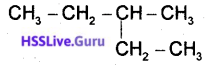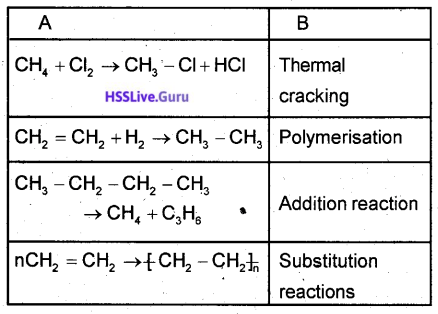# Kerala SSLC Chemistry Model Question Paper 1 English Medium

Students can Download Kerala SSLC Chemistry Model Question Paper 1 English Medium Pdf, Kerala SSLC Chemistry Model Question Papers helps you to revise the complete Kerala State Board New Syllabus and score more marks in your examinations.

## Kerala SSLC Chemistry Model Question Paper 1 English Medium

General Instructions:

1. The first 15 minutes is the cool off time. You may use the time to read and plan your answers.
2. Answer the questions only after reading the instructions and questions thoroughly.
3. Questions with marks series 1, 2, 3 and 4 are categorized as sections A, B, C and D respectively.
4. Five questions are given in each section. Answer any four from each section.
5. Answer each question by keeping the time.

Time: 1½ Hours
Total Score: 40 Marks

Section – A

(Answer any 4 questions from 1 to 5. Each question carries 1 score) (1 × 5 = 5)

Question 1.
The element with 1s2 2s2 2p6 3s2 3p6 is placed in the group of the periodic table.
a) 1
b) 6
c) 8
d) 18
18

Question 2.
The reaction taking place at the anode of a zinc – copper galvanic cell …………
a) Zn → Zn2+ + 2e
b) Zn2+ + 2e → Zn
c) Cu → Cu2+ + 2e
d) Cu2+ + 2e → Cu
2.
Zn → Zn<>sup>2+ + 2e

Question 3.
The metal that liberate hydrogen gas when react with steam is ………….
a) Magnesium
b) Zinc
c) Iron
d) Sodium
Iron

Question 4.
The substance used to remove moisture from ammonia is ……………….
b) Sulphuric acid
c) Calcium oxide
d) Silicon dioxide
Calcium oxide

Question 5.
The functional group present in the compound CH3 – O-CH3 is …………
a) Hydroxyl
b) Alkoxy
c) Methyl
d) Carboxylic
Alkoxy

Section – B

(Answer any 4 questions from 6 to 10. Each question carries 2 scores)(4 × 2 = 8)

Question 6.
Calculate the number of molecules in 90g of water. (Hint-Atomicmass H = 1 O = 16)
1 GMM of water = 18 g.
90 g water = $$\frac{90}{18}$$ = 5 mol
No. of molecules in 5 mol = 5 x 6.022 x 1023

Question 7.
The molecular mass of CO2 is 44.
a) Find out the number of molecules in 220g CO2.
a) 220g CO2 = $$\frac{224}{44}$$ = 5 mol
∴ No. of molecules = 5 x 6.022 x 1023
b) 5×22.4 = 112 L

b) What will be the volume of this much CO2 at STP?
(Hint: The molar volume of gases at STP = 22.4 L)

Question 8.
The equation of the industrial production of ammonia is given below.
N2(g) + 3H2(g) ⇌ 2NH3(g) + Heat
a) By which name the industrial production of ammonia is known?
b) What will be the effect of increased pressure in ‘ this reaction?
c) What will happen if ammonia is removed from the system at regular intervals?
a) Heber’s process
b) Due to forward reaction number of molecules decreases. So increase in pressure is favourable to forward reaction.
c) Forward reaction increases, more product will be formed.

Question 9.
Certain elements are arranged in the order as they appear in the electrochemical series.
Answer the questions that follows based on this Na > Mg > Al > Zn > Cu > Ag
a) Which of these element is likely to be found in free state in nature?
b) Which of these metal will produce the stablest compound?
c) Which of these metal is produced by reducing its ore with CO?
a) Ag
b) Na
c) Zn

Question 10.
The structural formula of an organic compound is given below.a) What is the total number of carbon atom in the longest carbon chain in this compound?
b) Write the name and position of the branch.
c) Write the IUPAC name of the compound.
a) 5
b) Methyl, 3
c) 3 – methyl pentane

Section – C

(Answer any 4 questions from 11 to 15. Each question carries 3 scores) (4 × 3 = 12)

Question 11.
The subshell electron configuration of certain elements are given below. (Symbols are not real)
A = [Ar] 4s2 3d1 B = [Ne]3s1
C = [Ar] 4s1 D = [Ne]3s23p5
a) Write any characteristic of the element A.
b) Which among these belong to the same group?
c) Which among these belong to the same period?
a) Shows variable oxidation states or forms coloured compounds.
b) B and C
c) C and D

Question 12.
Certain samples are given below.a) Find out the number of moles in each sample.
b) Arrange them in the increasing order of number of molecules.
(Hint: Atomic mass of H = 1, He = 4, Ca = 20)
200g H2 = $$\frac{200}{2}$$ = 100 mol
200 g He = $$\frac{200}{4}$$ = 50 mol
200 g Ga = $$\frac{200}{20}$$ = 10 mol
200g Ca < 200g He < 200g H2

Question 13.
a) Which substances are used as the anode,
cathode and electrolyte when copper is refined by electrolysis.
b) It is said that electrolytic refining of copper is economic. Why?
a) Anode – Impure copper (Copper to be purified)
Cathode – Pure Copper rod
Electrolyte – CuSO4 solution
b) When copper is purified by electrolysis the residue left behind at the anode, called anode mud, often contains precious metals like gold.

Question 14.
Match those given columns suitably.

 Metal Name of ore Chemical formula Aluminium Calamine Al2O3.2H2O Iron Bauxite ZnCO3 Zinc Haematite Fe2O3

Aluminium – Bauxite – Al2O3-2H2O
Iron – Haematite – Fe2O3
Zinc – Calamine – ZnCO3

Question 15.
There are compounds with same molecular formula but different structural formula,
a) Write two compounds with different structural formula and the molecularformulaC3H8O.
b) By what name these compounds are known?(1)
a) CH3-O-CH2-CH3
CH3-CH2-CH2-OH
b) Isomers

Section – D

(Answer any 4 questions from 16 to 20. Each question carries 4 scores)(4 × 4 = 16)

Question 16.
In the table given below, under A certain chemical reactions and under B types of chemical reactions are given. Match them suitably.CH4 + Cl2 → CH3 – Cl + HCl
– Substitution reaction

CH2 = CH2 + H2 → CH3 – CH3

CH3-CH2-CH2-CH3 → CH4-C3H6
– Thermal cracking

nCH2 = CH2 → [-CH2-CH2-]n
– Polymerisation

Question 17.
The chemical reactions taking place inside blast furnance during the manufacture of ‘iron is given below. Analyse it and answer the questions given below.
CaCO3(s) → CaO(s) + CO2(g)
CaO(s) + SiO2(s) → CaSiO3(s)
C(s) + O2(g) → CO2(g) + Heat
CO(g) + C(s) + Heat → 2CO(g)
Fe2O3(s) + 3CO(g) → 2Fe(s) + 3CO2(g)
a) Write the chemical formula of the ore of iron.
b) Which compound is the actual reducing agent in the reaction?
c) Which equation shows slag formation?
a) Fe2O3
b) CO
C) CaO(s) + SiO2(s) →CaSiO3(s)
d) FeO3(s) + 3CO(g) → 2Fe(s) + 3CO2(g)

Question 18.
The equation of a reversible reaction is given below.
2SO2(g) + O2(g) ⇌ 2SO3(g) + Heat
a) Write the equations of endothermic and exothermic reactions.
b) Write the mole ratio of the reactants and products
c) Suggest methods to get more product.
a) Endothermic
2SO3(g)+Heat → 2SO2(g +O2(g)
Exothermic
2SO2(g) + O2(g) → 2SO3(g)+Heat

b) 2:1:2
c) Increase the concentration of the reactants
Remove the product from the system
Decrease the temperature
Increase the pressure.

Question 19.
a) Sulphuric acid is known as ‘the king of chemicals’.
What is the basis for this?
b) How concentrated sulphuric acid is diluted in the laboratory?
c) The wooden cupboards in which concentrated sulphuric acid is often seen charred. Why?
a) Due to the wide range of uses sulphuriç acid is known as the king of chemicals eg: Used to make explosives, petroleum refining dehydrating agent, manufacture of chemical fertilizers, etc.
b) Take water in a beaker and add concentrated acid slowly along the side and stir continuously. Otherwise, the add will spurt over as the dissolution is exothermic.
c) Sulphuric absorbs hydrogen and oxygen present in substances in the ratio as that in water. So carbon only remains (dehydrating property)

Question 20.
a) How do galvanic cells and electrolytic cells differ each other?
b) Write any two practical uses of electrolysis.
a)

• Galvanic cells converts chemical energy in to electrical energy.
• Electrolytic cell converts electrical energy into chemical energy
• The anode in a galvanic cell is negative and cathode is positive.
• The anode in an electrolytic cell is positive and cathode is negative

b)

• Production of metal .
• Production of non metals
• Production of compounds
• Refining of metals
• Electroplating